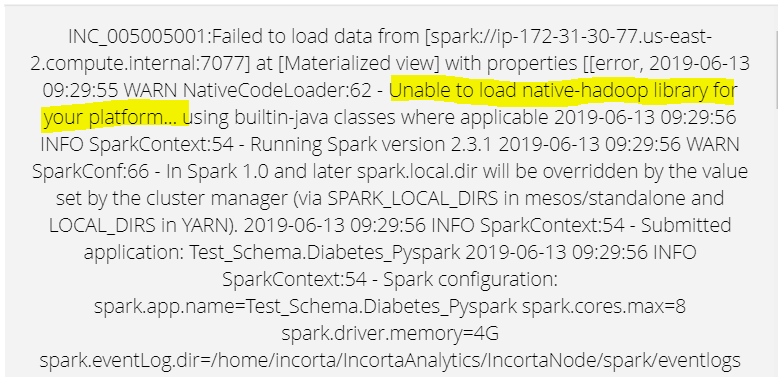1

# Python and PySpark librariesIn the materialized view, I'm attempting to import the python and pyspark libraries. But I get the error as in the image[native library can not be loaded ]. I have to use pyspark to conduct ML.

Is there a way to install packages that are dependent?

Note: my sample code:

import pyspark.sql.functions as F
import numpy as np
from pyspark.ml.feature import Imputer
from pyspark.sql.functions import when
raw_data.createOrReplaceTempView("raw_data")
import numpy as np
str1 = """SELECT * FROM raw_data"""
df1 = spark.sql(str1)
save(df1)

2replies Oldest first
• Oldest first